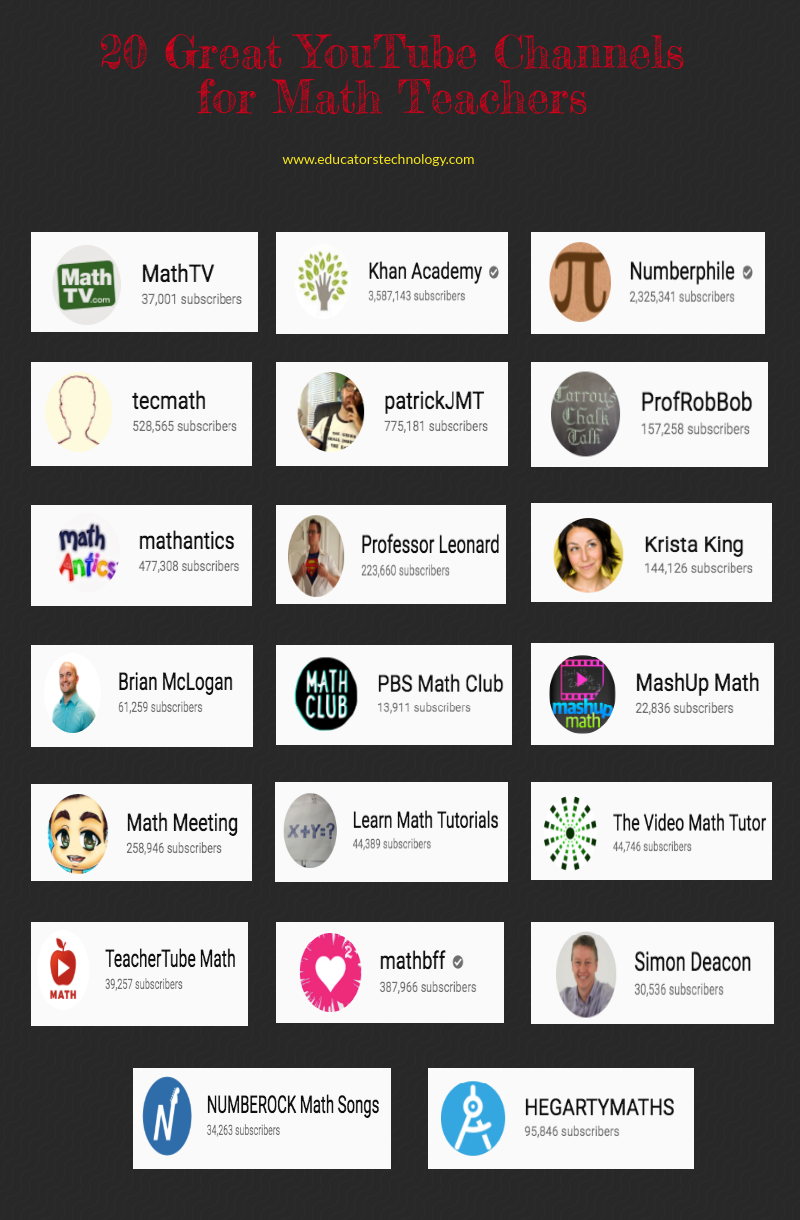# 20 Great YouTube Channels for Math Teachers

December 28, 2017
For those of you teaching math, here is a collection of some of the best YouTube channels we reviewed this year. You can draw on these resources to access and find educational video content to help you in the teaching of math for different grade levels. Some of these channels are set up and run by math teachers and others by popular math websites. You may want to go through the collection and see what works for you. Links to the channels are under the visual.1- Math TV
‘MathTV.com has over 10,000 instructional videos to help students learn everything from basic math through calculus. Each problem is worked by multiple instructors.’

This list would be incomplete without Khan Academy in it. The channel embeds math courses and video tutorials covering a large spectrum of math concepts.

3- Numberphile
This is another popular math channel on YouTube. It features math videos with a primary focus on numbers

4- Techmath
Tech math is a channel that  ‘obsesses over methods to make maths easier! [It provides] Math Tricks for fast results!’

5- PatrickJMT
This channel is run by a former math instructor and provides a wide variety of videos tackling different math problems.

6- ProfRobBob
‘Here you can find full length lessons with multiple examples covering topics from Algebra, Geometry, Algebra 2, Trigonometry, PreCalculus, Calculus, and Statistics.’

7- Mathantics
Another great channel for math teachers. Mathantics features tons of video tutorials on everything related to Math.

8-  Professor Leonard
‘This Channel is dedicated to quality mathematics education. It is absolutely FREE so Enjoy! Videos are organized in playlists and are course specific.’

9- Krista King
Krista ‘recorded tons of videos and written out cheat-sheet style notes and formula sheets to help every math student—from basic middle school classes to advanced college calculus—figure out what’s going on, understand the important concepts, and pass their classes, once and for all.’

10- Free Math Videos
This channel is also run by a math teacher who offers free math tutoring and videos to help students ‘gain better understanding of mathematics’.

11- PBS Math Club
PBS Math Club  'cover the 7th grade Common Core Standard for math; topics like adding and subtracting integers, equations, ratios and proportions, and statistics.'

12- Mashup Math
‘MashUp Math, a mathematics education YouTube channel run by young teachers on a mission to meet the unique needs of students with a visual learning preference.

13- Math Meeting
Math Meeting covers almost all ‘math subjects from basic level math through upper level calculus and statistics’.

14- Learn Math Tutorials
‘This channel provides a growing resource of helpful math tutorials.’

15- The Video Math Tutor
The Video Math Tutor offers a variety of math tutoring videos ‘plus many helpful study tips, calculator tips, and "Brain Teasers."’

16- TeacherTube Math
Another great math channel that covers topics such as fractions, multiplication, elementary math, middle school math, high school math, college math, and anymore.

17- Mathbff
This channel offers math videos covering algebra and calculus from a MIT graduate.

18- Simon Deacon
‘This channel is to help with your math studies through school, college and university. Real maths, tips, resources, videos, word problems, numeracy, arithmetic, games, BIG questions, past papers are all either on the channel or the Maths Wrap website.’

19- NumberRock Math Songs
This channels provides animated math videos and educational songs for kids to make math learning fun and engaging.

20- HEGARTY Maths
‘This channel is to provide help to students out there who need a little extra help and cannot afford tutoring. I produce the channel for free in my free time outside my full time job as a maths teacher.’